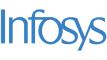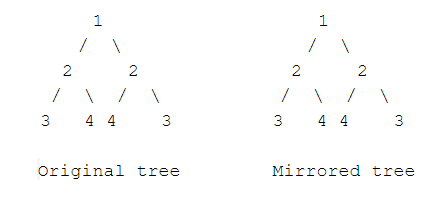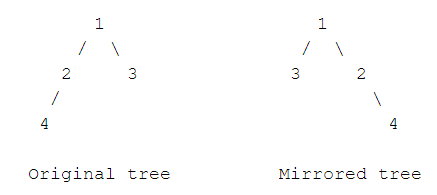New update is available. Click here to update.
Topics

# Symmetric Tree

Easy0/40
Average time to solve is 20m+13 more companies

## Problem statement

You are given a binary tree, where the data present in each node is an integer. You have to find whether the given tree is symmetric or not.

Symmetric tree is a binary tree, whose mirror image is exactly the same as the original tree.

For Example:Detailed explanation ( Input/output format, Notes, Images )
Constraints:
``````0 <= N <= 10^5
1 <= Data <= 10^5

Where 'N' denotes the number of nodes in the given binary tree and 'Data' denotes the node value.

Time limit: 1sec
``````
Sample Input 1:
``````1 2 2 3 4 4 3 -1 -1 -1 -1 -1 -1 -1 -1
``````
Sample Output 1:
``````Symmetric
``````
Explanation For Sample 1:
``````This is a symmetric tree:
``````Sample Input 2:
``````1 2 3 4 -1 -1 -1 -1 -1
``````
Sample Output 2:
``````Asymmetric
``````
Explanation For Sample 2:
``````This is an asymmetric tree:
``````Console